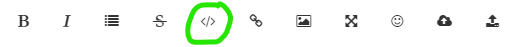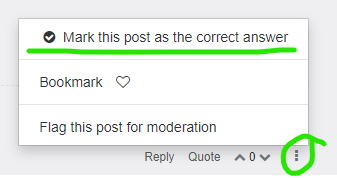# how to get object's position and material color on all time line?

• Dear. MAXON's SDK Team

I use R21 on microsoft windows with python.

I have threads that how to get object's position and material color on all time line.
below my simple source code.

``````import c4d							# import Cinema 4D module
doc = c4d.documents.GetActiveDocument()	# Cinema 4D active document

number_of_sphere = 10		# [Set] sphere number
frames_count = 2600		# [Set] frames counter

for i in range(0, number_of_sphere):
for f in range(0, frames_count):
doc.SetTime(c4d.BaseTime(f, doc[c4d.DOCUMENT_FPS]))
obj = doc.SearchObject('Sphere_' + str(i))
# get object position
x = int(obj.GetMg().off.x)
y = int(obj.GetMg().off.y)
z = int(obj.GetMg().off.z)
# get object color
r = int(obj[c4d.ID_BASEOBJECT_COLOR].x * 255)
g = int(obj[c4d.ID_BASEOBJECT_COLOR].y * 255)
b = int(obj[c4d.ID_BASEOBJECT_COLOR].z * 255)
print(obj.GetName() + ", Frame = " + str(f) + ", (X,Y,Z) = " + str(x) + "," + str(y) + "," + str(z) + ", RGB = " + str(r) + "," + str(g) + "," + str(b))
``````

Please, guide to me for method of this.

Cheers,
MAXON's SDK Team

• Hi @jhpark!
I don't work for the SDK team, but I believe the script below will do what you want.

1. When entering your code into a post on this forum, make sure you hit this button firstIt creates code tags in your post. Put your code in between those and then it will format your code automatically.

2. Also, after submitting, hit the button Topic Tools at the bottom right of your post to Ask as Question.3. When someone has answered your question correctly, click this button at the bottom of their post.This makes it clear to the moderators when the question has been correctly answered.

Here's the code. Because you were using `ID_BASEOBJECT_COLOR`, I was unsure if you wanted the object's display color or the material color (they are two different things), but I wrote this for the sphere's texture tags' material's color. Also, the code is for the spheres' relative position. More work would need to be done to get the animating position track values into global space.

``````import c4d
from c4d import gui

def GetNextObject(op):
#function for navigating the hierarchy
if op==None: return None
if op.GetDown(): return op.GetDown()
while not op.GetNext() and op.GetUp():
op = op.GetUp()
return op.GetNext()

def getPreviewRange(doc,fps):
#returns the active preview range
fps = doc.GetFps()
fromTime = doc.GetLoopMinTime().GetFrame(fps)
toTime = doc.GetLoopMaxTime().GetFrame(fps)+1
return [fromTime,toTime]

def convertVecToRgb(vector):
#converts vector to rgb list
return [vector*255,vector*255,vector*255]

def main(doc):
fps = doc.GetFps()
previewRange = getPreviewRange(doc,fps) #rather than needing to set frames manually, you can simply resize your preview range.
frame_count = previewRange-previewRange

# this section navigates the hierarchy and saves all of the spheres to a list called 'output'
# it's better to do this than to use doc.SearchObject in the case you have multiple spheres with the same name
obj = doc.GetFirstObject()
if obj==None:
gui.MessageDialog('There are no objects in the scene.')
return

output = []
while obj and obj!=None:
if obj.GetType() == c4d.Osphere:
output.append(obj)
obj = GetNextObject(obj)

if len(output) == 0:
gui.MessageDialog('There are no spheres in the scene.')

# loops through spheres in the scene
for sphere in output:
#prints a separating line to the console
print '#' * 80
for f in range(previewRange, previewRange):
doc.SetTime(c4d.BaseTime(0, doc[c4d.DOCUMENT_FPS]))
keyTime = c4d.BaseTime(f,fps) #get the current frame

# POSITION
pTracks = sphere.GetCTracks() #get the sphere's animating tracks

pos = [sphere.GetMl().off.x,sphere.GetMl().off.y,sphere.GetMl().off.z] #get the sphere's default relative position

#replace those values with the animating ones.
for t in pTracks:
descid = t.GetDescriptionID() #get the track's id
if descid.id == c4d.ID_BASEOBJECT_REL_POSITION: #see if it matches the object's position track
curve = t.GetCurve() #get the track's animation curve
keyvalue = curve.GetValue(keyTime, fps) #get the animation curve's value at the current frame
if descid.id == c4d.VECTOR_X:
pos = keyvalue #add to x
elif descid.id == c4d.VECTOR_Y:
pos = keyvalue #add to x
elif descid.id == c4d.VECTOR_Z:
pos = keyvalue #add to z

# MATERIAL COLOR
tags = sphere.GetTags() #get sphere's tags

matColor = [] #create material color list

for tag in tags: #loop through sphere's tags
if tag.GetType() == c4d.Ttexture: #check if tag is a texture tag
mat = tag.GetMaterial() #if yes, get the tag's material

tracks = mat.GetCTracks() #get the material's animating tracks

for t in tracks:
descid = t.GetDescriptionID() #get the track's id
if descid.id == c4d.MATERIAL_COLOR_COLOR: #see if it matches the material color track
curve = t.GetCurve() #get the track's animation curve
keyvalue = curve.GetValue(keyTime, fps) #get the animation curve's value at the current frame

if len(tracks) == 0: #in case it's not animating, use general Color
matColor = convertVecToRgb(mat[c4d.MATERIAL_COLOR_COLOR])

# I prefer using string formatting with the placeholder %s for strings, %d for numbers,
# and the % as the replacement operator
print("Name: %s, Frame: %d, Position (x,y,z): %d,%d,%d, Material Color (r,g,b): %d,%d,%d"%(
sphere.GetName(),f,pos,pos,pos,
matColor,matColor,matColor))

if __name__=='__main__':
# rather than using documents.GetActiveDocument, I found that you can pass a reference to the document using this method
main(doc)
``````

Here's a scene file where the object's display colors and material colors are different. The display colors are visible in the viewport, but you will see the material color if you render.
Spheres.c4d

• Hi jhpark, thanks for reaching out us.

Aside from the notes left by @blastframe - thanks dude for the remarks - I think it's worthy, thinking of a more generic scene, to mention the need BaseDocument::ExecutePasses() to be sure that everything is actually evaluated before querying the scene rather than the EventAdd() which serves a different scope.
This function is responsible to execute the scene evaluation and, consequently to be sure that, moving from a frame to another, all the items in the scene reflect the changes imposed by the frame switch.

The approach used by @blastframe actually operates on CTracks and key but, although this approach works fine for your specific case, when more evaluation dependencies are created in the scene you could easily end up in unexpected results.

The code could then look like

``````    frames_count = 10 # [Set] frames counter

for f in range(0, frames_count):
doc.SetTime(c4d.BaseTime(f, doc.GetFps()))

# evaluate the scene
doc.ExecutePasses(None, True, True, True, c4d.BUILDFLAGS_NONE)

obj = doc.SearchObject('Cube')

# get object position
x = int(obj.GetMg().off.x)
y = int(obj.GetMg().off.y)
z = int(obj.GetMg().off.z)

# get object color
r = int(obj[c4d.ID_BASEOBJECT_COLOR].x * 255)
g = int(obj[c4d.ID_BASEOBJECT_COLOR].y * 255)
b = int(obj[c4d.ID_BASEOBJECT_COLOR].z * 255)
print("Frame = " + str(f) + ", (X,Y,Z) = " + str(x) + "," + str(y) + "," + str(z) + ", RGB = " + str(r) + "," + str(g) + "," + str(b))

``````